• 在很多场景的计算中，最终得到的数值例如123.45678，要截取2小数得到123.45，而不是默认的四舍五入方法得到123.46，如何实现呢？ 一.小数点后取2位四舍五入）的方法 ...小数点后取2位四舍五入...

在很多场景的计算中，最终得到的数值例如123.45678，要截取2位小数得到123.45，而不是默认的四舍五入方法得到123.46，如何实现呢？

文章目录一.小数点后取2位（四舍五入）的方法方法一：round（）函数方法二：'%.2f' %f 方法方法三：Decimal（）函数二.小数点后取2位（四舍五不入）的方法方法一：
一.小数点后取2位（四舍五入）的方法
方法一：round（）函数

其实这个方法不推荐大家使用，查询资料发现里面的坑其实很多，python2和python3里面的坑还不太一样，在此简单描述一下python3对应的坑的情况。

a = 1.23456
b = 2.355
c = 3.5
d = 2.5
print(round(a, 3))
print(round(b, 2))
print(round(c))
print(round(d))

结果：
1.235	# 1.23456最终向前进位了
2.35	# 2.355居然没进位
4		# 最终3.5居然变为4了
2		# 最终2.5取值变为2


（1）通过上面的函数，看着是不是很晕，感觉round（x,n）函数是否进位也没看出是啥规律
（2）round（x,n）函数中，是否进位或四舍五入，取决于n位以及n+1位小数的值
（3）只有当n+1位数字是5的时候，容易混淆，如果n为偶数，则n+1位数是5，则进位，例如round（1.23456，3）最终变为1.235
（4）如果n为奇数，则n+1位是数5，那不进位，例如round（2.355，2），最终为2.35
（5）如果n为0，即没有填写n的时候，最终结果与上面相反，即整数部分为偶数的时候，小数位5不进位，例如（round（2.5）变为2）。
（6）整数部分为奇数的时候，小数位5进位。（round（3.5）变为4）

看完如上的部分，感觉是不是更晕了，所以round（）不推荐使用，目前也不知道设计这个函数的目的在哪里？有谁知道麻烦告知一下？

方法二：’%.2f’ %f 方法
﻿﻿f = 1.23456

print('%.4f' % f)
print('%.3f' % f)
print('%.2f' % f)

结果：
1.2346
1.235
1.23


（1）原本以为：这个方法是最常规的方法，方便实用，居家旅行必备！
（2）但是…

f = 0.625
print('%.2f' % f)

# 结果：0.62


具体是否进位，有个概率问题，感兴趣的朋友可以看看评论里面的信息。
感谢weixin_43094430这位朋友的提示，也感谢其他朋友的参与

方法三：Decimal（）函数
from decimal import Decimal

aa = Decimal('5.026').quantize(Decimal('0.00'))
bb = Decimal('3.555').quantize(Decimal('0.00'))
cc = Decimal('3.545').quantize(Decimal('0.00'))

print(aa)
print(bb)
print(cc)

结果：
5.03
3.56
3.54


decimal这个模块在很少用，如上图中，3.555结果为3.56，而3.545结果变为3.54，一个5进位了，一个是5没进位，具体原因不详。
所以不推荐使用这个方法！！！

二.小数点后取2位（四舍五不入）的方法

通过计算的途径，很难将最终结果截取2位，我们直接想到的就是如果是字符串，直接截取就可以了。
例如

num = '1234567'		#字符串num
print(num[:3])

结果：
123


如果是123.456取2位小数（截取2位小数），值需要把小数点右边的当做字符串截取即可
partition()函数（将字符串根据字符串切割）：
http://www.runoob.com/python/att-string-partition.html

num = '123.4567'
num_str = num.partition(".")
print(num_str)

结果：
('123', '.', '4567')   # 三个元素的元祖


拼接字符串：format（）函数的使用
https://blog.csdn.net/i_chaoren/article/details/77922939

方法一：
def get_two_float(f_str, n):
a, b, c = f_str.partition('.')
c = c[:n]
return ".".join([a, c])

num = "123.4567"		#（1）隐患一，传入函数的是字符串
print(get_two_float(num, 2))

num2 = '123.4'			# （2）隐患二，如果传入的字符串小数位小于最终取的位数
print(get_two_float(num2, 2))

结果：
123.45
123.4

最终版本：
def get_two_float(f_str, n):
f_str = str(f_str)      # f_str = '{}'.format(f_str) 也可以转换为字符串
a, b, c = f_str.partition('.')
c = (c+"0"*n)[:n]       # 如论传入的函数有几位小数，在字符串后面都添加n为小数0
return ".".join([a, c])

num = 123.4567
print(get_two_float(num, 2))

num2 = 123.4
print(get_two_float(num2, 2))

结果：
123.45
123.40



展开全文• 小数点后取2位四舍五入）的方法 方法一：round（）函数 其实这个方法推荐大家使用，查询资料发现里面的坑其实很多，python2和python3里面的坑还太一样，在此简单描述一下python3对应的坑的情况。 结果： ...
一.小数点后取2位（四舍五入）的方法

方法一：round（）函数
其实这个方法不推荐大家使用，查询资料发现里面的坑其实很多，python2和python3里面的坑还不太一样，在此简单描述一下python3对应的坑的情况。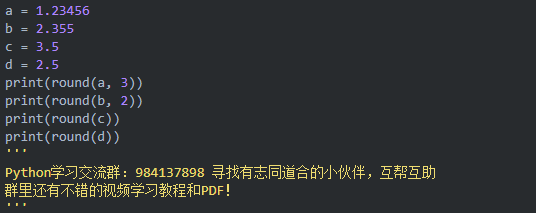结果：

1.235 # 1.23456最终向前进位了
2.35 # 2.355居然没进位
4 # 最终3.5居然变为4了
2 # 最终2.5取值变为2
（1）通过上面的函数，看着是不是很晕，感觉round（x,n）函数是否进位也没看出是啥规律
（2）round（x,n）函数中，是否进位或四舍五入，取决于n位以及n+1位小数的值
（3）只有当n+1位数字是5的时候，容易混淆，如果n为偶数，则n+1位数是5，则进位，例如round（1.23456，3）最终变为1.235
（4）如果n为奇数，则n+1位是数5，那不进位，例如round（2.355，2），最终为2.35
（5）如果n为0，即没有填写n的时候，最终结果与上面相反，即整数部分为偶数的时候，小数位5不进位，例如（round（2.5）变为2）。
（6）整数部分为奇数的时候，小数位5进位。（round（3.5）变为4）
看完如上的部分，感觉是不是更晕了，所以round（）不推荐使用，目前也不知道设计这个函数的目的在哪里？有谁知道麻烦告知一下？

方法二：’%.2f’ %f 方法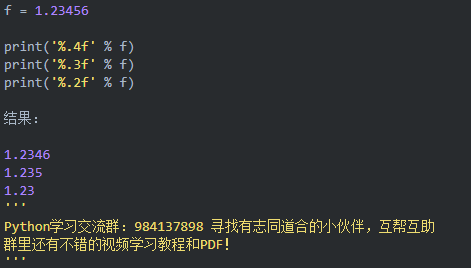（1）这个方法是最常规的方法，方便实用，居家旅行必备！
方法三：Decimal（）函数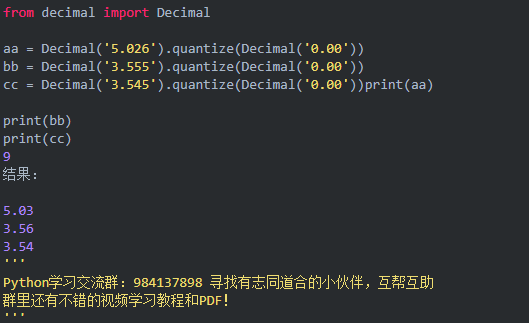decimal这个模块在很少用，如上图中，3.555结果为3.56，而3.545结果变为3.54，一个5进位了，一个是5没进位，具体原因不详。
所以不推荐使用这个方法！！！

二.小数点后取2位（四舍五不入）的方法

通过计算的途径，很难将最终结果截取2位，我们直接想到的就是如果是字符串，直接截取就可以了。
例如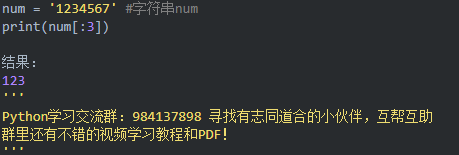如果是123.456取2位小数（截取2位小数），值需要把小数点右边的当做字符串截取即可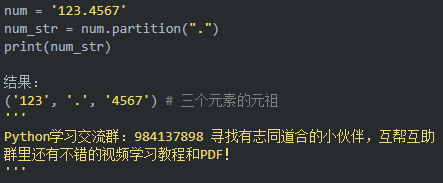方法一：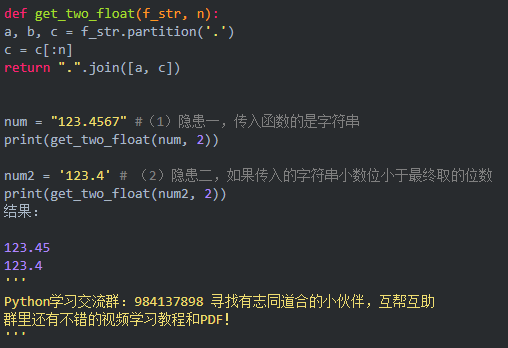最终版本：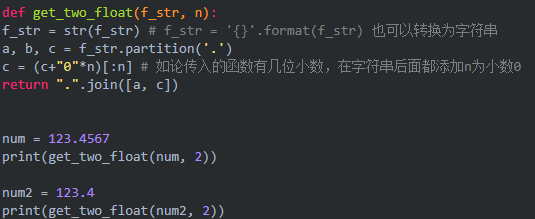展开全文• 小数点后取2位四舍五入）的方法方法一：round（）函数其实这个方法推荐大家使用，查询资料发现里面的坑其实很多，python2和python3里面的坑还太一样，在此简单描述一下python3对应的坑的情况。 a = 1.23456b ...
一.小数点后取2位（四舍五入）的方法方法一：round（）函数其实这个方法不推荐大家使用，查询资料发现里面的坑其实很多，python2和python3里面的坑还不太一样，在此简单描述一下python3对应的坑的情况。
a = 1.23456b = 2.355c = 3.5d = 2.5print(round(a, 3))print(round(b, 2))print(round(c))print(round(d))结果：
1.235   # 1.23456最终向前进位了2.35    # 2.355居然没进位4       # 最终3.5居然变为4了2       # 最终2.5取值变为2（1）通过上面的函数，看着是不是很晕，感觉round（x,n）函数是否进位也没看出是啥规律（2）round（x,n）函数中，是否进位或四舍五入，取决于n位以及n+1位小数的值（3）只有当n+1位数字是5的时候，容易混淆，如果n为偶数，则n+1位数是5，则进位，例如round（1.23456，3）最终变为1.235（4）如果n为奇数，则n+1位是数5，那不进位，例如round（2.355，2），最终为2.35（5）如果n为0，即没有填写n的时候，最终结果与上面相反，即整数部分为偶数的时候，小数位5不进位，例如（round（2.5）变为2）。（6）整数部分为奇数的时候，小数位5进位。（round（3.5）变为4）看完如上的部分，感觉是不是更晕了，所以round（）不推荐使用，目前也不知道设计这个函数的目的在哪里？有谁知道麻烦告知一下？
方法二：’%.2f’ %f 方法f = 1.23456
print('%.4f' % f)print('%.3f' % f)print('%.2f' % f)结果：
1.23461.2351.23（1）这个方法是最常规的方法，方便实用，居家旅行必备！方法三：Decimal（）函数from decimal import Decimal
aa = Decimal('5.026').quantize(Decimal('0.00'))bb = Decimal('3.555').quantize(Decimal('0.00'))cc = Decimal('3.545').quantize(Decimal('0.00'))print(aa)
print(bb)print(cc)9结果：
5.033.563.54decimal这个模块在很少用，如上图中，3.555结果为3.56，而3.545结果变为3.54，一个5进位了，一个是5没进位，具体原因不详。 所以不推荐使用这个方法！！！
二.小数点后取2位（四舍五不入）的方法通过计算的途径，很难将最终结果截取2位，我们直接想到的就是如果是字符串，直接截取就可以了。 例如
num = '1234567'     #字符串numprint(num[:3])
结果：123如果是123.456取2位小数（截取2位小数），值需要把小数点右边的当做字符串截取即可 partition()函数（将字符串根据字符串切割）： http://www.runoob.com/python/att-string-partition.html
num = '123.4567'num_str = num.partition(".")print(num_str)
结果：('123', '.', '4567')   # 三个元素的元祖拼接字符串：format（）函数的使用 https://blog.csdn.net/i_chaoren/article/details/77922939
方法一：def get_two_float(f_str, n):    a, b, c = f_str.partition('.')    c = c[:n]    return ".".join([a, c])
num = "123.4567"        #（1）隐患一，传入函数的是字符串print(get_two_float(num, 2))
num2 = '123.4'          # （2）隐患二，如果传入的字符串小数位小于最终取的位数print(get_two_float(num2, 2))结果：
123.45123.4最终版本：
def get_two_float(f_str, n):    f_str = str(f_str)      # f_str = '{}'.format(f_str) 也可以转换为字符串    a, b, c = f_str.partition('.')    c = (c+"0"*n)[:n]       # 如论传入的函数有几位小数，在字符串后面都添加n为小数0    return ".".join([a, c])
num = 123.4567print(get_two_float(num, 2))
num2 = 123.4print(get_two_float(num2, 2))
转载于:https://www.cnblogs.com/klb561/p/10029946.html
展开全文• 测试代码如下： double dayDiff2 = 5.06135778; BigDecimal usedM = new BigDecimal(dayDiff2);... //保留两小数且四舍五入 usedM = usedM.setScale(2, BigDecimal.ROUND_HALF_UP); 测试结果如图： ...
   测试代码如下：

double dayDiff2 = 5.06135778;
BigDecimal usedM = new BigDecimal(dayDiff2);
//保留两位小数且四舍五入
usedM = usedM.setScale(2, BigDecimal.ROUND_HALF_UP);

测试结果如图：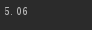展开全文• 碰到一个这样的问题：对于一个变量（单位是元），需要转成（万元）之后保留1小数，且对1小数后面的值需要进行四舍五入，不是截取到1小数；如：15500元==>1.6万元 需要在jsp中处理，网上查了一下，发现使用...jstl jsp
• * 保留double类型小数不四舍五入，直接小数 比如：10.1269 返回：10.12 * * @param doubleValue * @return */ public static String calculateProfit(double doubleValue) { // 保留4小数 ...
• c# 取二位小数点，四舍五入2009-08-09 15:01double d = 0.12545678; Console.WriteLine(d); Console.WriteLine(d.ToString("F2")); //0.13 Console.WriteLine(d.ToString("N2")); //0.13 Console.Read(); double...
• 小数，排除非数字类型的情况下，直接使用.toFixed(n) Number.toFixed(n) -- ...如果不要四舍五入，并且一定要去2位小数，即使是“12.1”这种要变成“12.10”可以保留3小数，然后截取的方式，截取前面2位
• java 小数点 不四舍五入,怎么做 正常版: //正常版: import java.text.DecimalFormat; import java.math.RoundingMode; DecimalFormat formater = new DecimalFormat(); formater....
• 功能：将浮点数四舍五入小数点后2位 用法：changeTwoDecimal(3.1415926) 返回 3.14 changeTwoDecimal(3.1475926)返回 3.15 js保留2位小数（强制） 对于小数点位数大于2位的，用上面的函数没...
• 一、四舍五入取整数Math.round() console.log(Math.round(2.5));//3 console.log(Math.round(2.4));//2 <script> function myFunction(){ document.getElementById("demo").innerHTML=Math.round(2.5); }...
• function formatFloat1(src, pos) {  return Math.round(src*Math.pow(10, pos))/Math.pow(10, pos);... return ""+src.replace(/^(.*/..{2}).*$/,"$1")// 小数点  }  return eval(src).toFixed(2); }javascript function
• 如图 打印结果 toStringAsFixed(3)这个方法会进行四舍五入，所以我们必须3，防止第二位四舍五入，再进行截取即可
• Java四舍五入保留1小数的方法： import java.math.BigDecimal; public class Test { public static void main(String[] args) { double d1 = 3.02; System.out.println(d1); //利用字符串格式化的...java
• 保留两小数（不四舍五入）方法： public static String calculateProfit(double doubleValue) { // 保留4小数 DecimalFormat df = new DecimalFormat("0.0000"); String result = df.format(doubleValue); ...
• 按照指定的小数进行四舍五入之后返回一个数值，比如： select round(31.1415) from dual; --返回 31 ，传参数，默认保留整数 select round(31.1415,2) from dual; --返回 31.14 select round(31.14155,3) from ...ROUND函数
• 保留小数点两 不四舍五入 Output：0.66 echo bcdiv(2,3,3); 2/3 =0.666... 保留小数点三 不四舍五入 Output：0.666 echo bcdiv(2,3,4); 2/3 =0.6666.... 保留小数点四 不四舍五入 Output：...
• //例如小数3 double a=1.1234567； int index = a.ToString().IndexOf(".");//小数点所在位置索引 if (index + 1 + n > a.ToString().Length|| a.ToString().IndexOf(".")==-1)//索引大于长度或a为整数 ...
• js中小数向上取整数，向下取整数，四舍五入取整数的实现。 1.ceil() 向上 2.floor() 向下 3.round() 四舍五入 直接看下面的例子，结果一目了然。代码下面的是对应的打印结果。 Math.floor(3/4) 0 Math.floor(1/4) 0 ...向下取整数
• 对x进行四舍五入保留n小数： (Math.round(x * 10^n) / (10^n)).toFixed(n); 10的n次方在这里写成10^n只是为了方便表达，js中应该使用Math.pow(10,n)或1en。 2.解释 Math.round：把一个数字舍入为它最接近的...JS
• php保留两小数并且四舍五入  Php代码  $num = 123213.666666;...php保留两小数并且不四舍五入 Php代码$num = 123213.666666;  echo sprintf("%.2f",substr(sprin
• // 案例：四舍五入保留小数点1 double d1 = 123.456; double d2 = 654.321; double v1 = (double) Math.round(d1 * 10) / 10; double v2 = (double) Math.round(d2 * 10) / 10; System.out...
• Java截取小数点随意几数，四舍五入和非四舍五入 Java中截取小数点随意几数，非四舍五入，直接截取； 比如：12.23467直接截取小数点后2位为12.23，直接截取小数点3为12.234 Java中截取小数点随意几数...Java
• php保留两小数并且四舍五入 ： $num = 123213.666666;...php保留两小数并且不四舍五入：$num = 123213.666666; echo sprintf("%.2f",substr(sprintf("%.3f", $num), 0, -2)); php进一法取整 ： • 计算个人所得税,需要四舍五入保留2位小数 csdn有很多篇文章讲述了四舍五入保留2位小数的多种方法,经过实测,下面这种方法最准确 通过对多个边缘值的测试,均无问题 public class MathUtil { private MathUtil() {... • php保留两小数并且... php保留两小数并且不四舍五入 代码如下:$num = 123213.666666; echo sprintf("%.2f",substr(sprintf("%.3f", \$num), 0, -2)); php进一法取整 代码如下:echo ceil(4.......